## Area of Kites

There are few windy-day pastimes more fun than flying a kite. Whether you prefer diamond kites, fighter kites, or Hello Kitty kites (they're all the rage now), flying them can make dull windy days infinitely more enjoyable.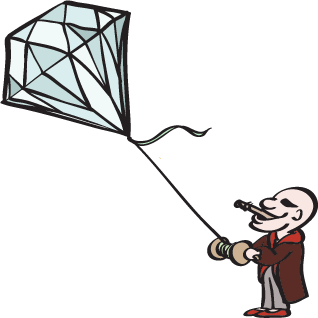The kites we're referring to probably aren't the ones that David Tomlinson was singing about. Oh well.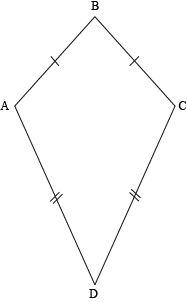We already know that a kite is defined as a quadrilateral that has two adjacent pairs of congruent sides. If all four sides of a kite are congruent, then we just have a rhombus. That's all good and fine, but it doesn't seem like any of that will help us find the area.

But wait. If we can use the diagonals of a rhombus to find the area, can we do the same with kites? If the two diagonals of a kite are perpendicular to each other (which they are), then we should be able to use the same type of formula.

Let's take a look at this logically for a second. Diagonals split a kite up into two pairs of congruent right triangles, each with a base of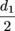, half of the horizontal diagonal.

We can split the vertical diagonal into two sections as well, but we don't know exactly what ratio. We'll just call the pieces x and y such that d2 = x + y. Since the area of the kite is the area of the four triangles combined, we can just add up the areas of the individual triangles.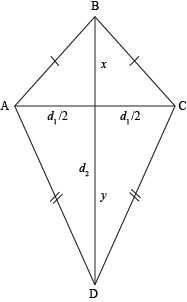A = 2(½bh1) + 2(½bh2)
A = bh1 + bh2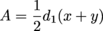Since we defined x and y so that x + y = d2, we can replace the quantity in parenthesis with the second diagonal. That means our final area formula for a kite ends up being relatively simple.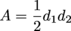### Sample Problem

Your fiancé would like you to buy her a \$20,000 diamond with a cross-section in the shape of a kite. She requests the diamond be a perfect cut, with diagonals of 3 mm and 5 mm. What is the area of the diamond's cross-section?

All we need to do is apply the formula for the area of a kite. We have the two diagonals, and that's all we need, right?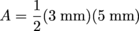A = 7.5mm2

Sure, that's all we need. Even still, a couple thousand bucks wouldn't hurt.

### Sample Problem

What is the area of a kite with diagonals of length 6 and 4?

To find the area of a kite, we just need the length of the two diagonals.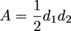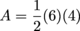A = 12 units2

Kites can get pretty complicated, but don't get tangled. They're not all easy breezy.

### Sample Problem

One diagonal of a kite is three times as long as the other. The shorter diagonal splits the longer one so that two isosceles right triangles are formed. If the area of the kite is 37.5 cm2, what is its perimeter?

Welp, we know that we have to use the area formula, but we should relate the two diagonals to each other first. If d1 is a certain length x, then d2 is three times that length, 3x. Plugging in these values to the area formula should give us the lengths of the diagonals.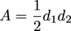37.5 = ½(x)(3x)
75 = 3x2
x2 = 25
x = 5

That means d1 is 5 cm and d2 is 3(5) = 15 cm long. Does that help us find the perimeter? Sort of, but we need to use the other piece of information that we're given: the shorter diagonal splits the longer diagonal to make two isosceles right triangles. It may help to draw a picture.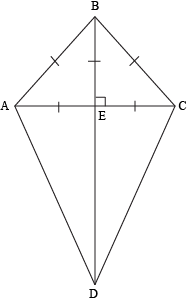The perimeter is the sum of all the lengths of the sides, AB + BC + CD + DA. Luckily, our life is made easier since AB = BC and CD = DA. That, and the fact that these segments are all hypotenuses of four right triangles.

The question tells us ΔABE and ΔCBE are congruent isosceles right triangles. In other words, AECEBE. We know that AC is 5 cm long, which means AE = CE = BE = 2.5 cm. This also means that DB = 15 cm (since the long diagonal is three times longer than the short one), and that DE = 15 – 2.5 = 12.5 cm.

If we remember our special triangles or the Pythagorean Theorem, we can find that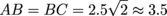. As for CD and DA, we can use the Pythagorean Theorem too, where a = 2.5 and b = 12.5.

a2 + b2 = c2
2.52 + 12.52 = c2
c ≈ 12.75

Therefore, CD = DA ≈ 12.75 cm. We can find the perimeter of the kite by adding all the side lengths together.

P = AB + BC + CD + DA
P = 3.5 + 3.5 + 12.75 + 12.75
P = 7 + 25.5
P = 32.5 cm

That's all we have to know when it comes to the areas of kites. Now it's time to pick one up and get flying. Kites, ho!